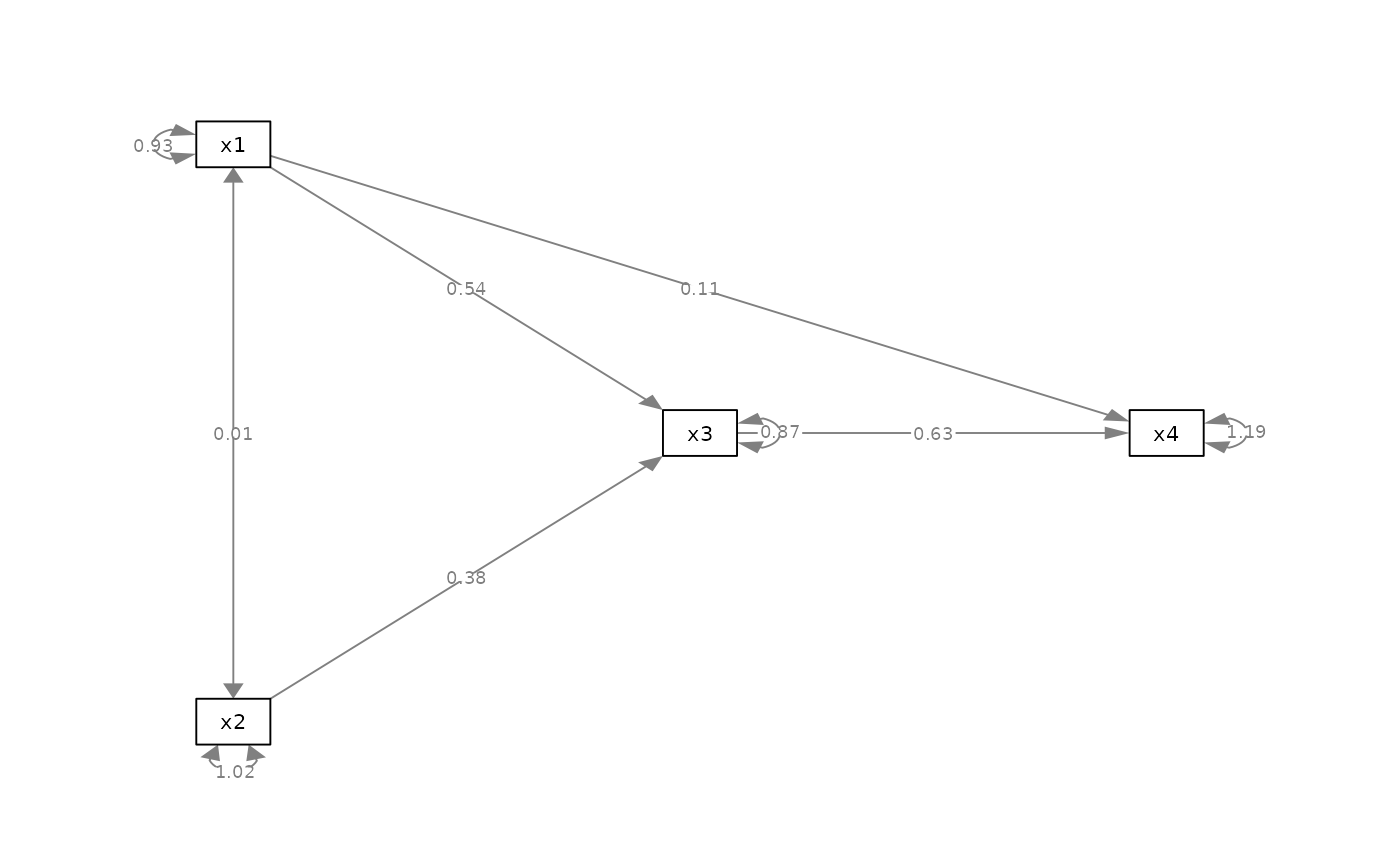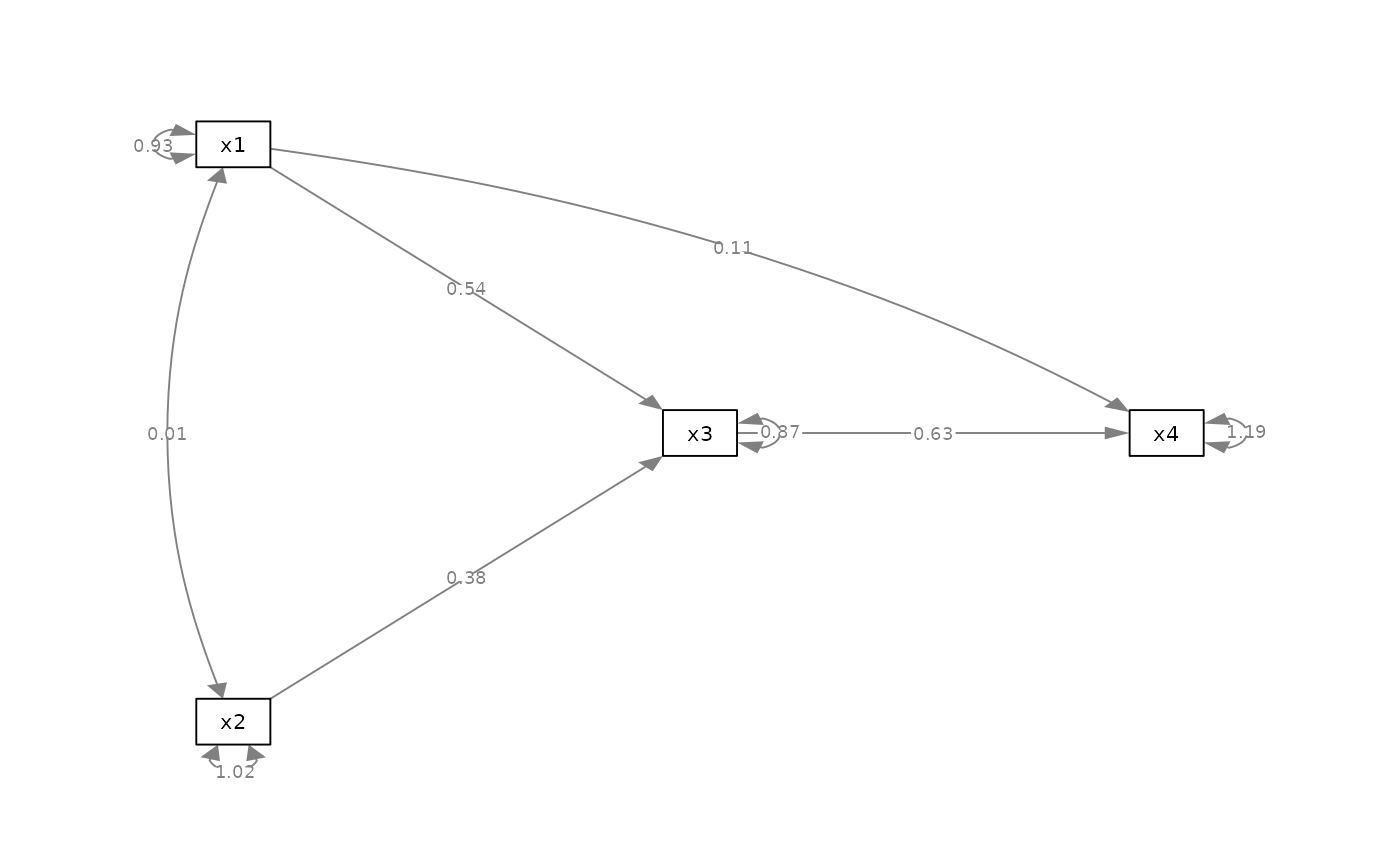Set the curve attributes of selected edges.

set_curve(semPaths_plot, curve_list = NULL)

## Arguments

semPaths_plot

A qgraph::qgraph object generated by semPlot::semPaths, or a similar qgraph object modified by other semptools functions.

curve_list

A named vector or a list of named list. For a named vector, the name of an element should be the path as specified by lavaan::model.syntax or as appeared in lavaan::parameterEstimates(). For example, to change the curve attribute of the path regressing y on x, the name should be "y ~ x". To change the curve attribute of the covariance between x1 and x2, the name should be "x1 ~~ x2". For example, c("y ~ x1" = -3, "x1 ~~ x2" = 2) change the curve attributes of the path from x1 to y and the covariance between x1 and x2 to -3 and 2, respectively. The order of the two nodes may matter for covariances. Therefore, if the curve of a covariance is not changed, try switching the order of the two nodes. For a list of named lists, each named list should have three named values: from, to, and new_curve. The curve attribute of the edge from from to to will be set to new_curve.

## Value

A qgraph::qgraph based on the original one, with curve attributes for selected edges changed.

## Details

Modified a qgraph::qgraph object generated by semPlot::semPaths and change the curve attributes of selected edges.

## Examples

mod_pa <-
'x1 ~~ x2
x3 ~  x1 + x2
x4 ~  x1 + x3
'
fit_pa <- lavaan::sem(mod_pa, pa_example)
lavaan::parameterEstimates(fit_pa)[, c("lhs", "op", "rhs", "est", "pvalue")]
#>   lhs op rhs   est pvalue
#> 1  x1 ~~  x2 0.005  0.957
#> 2  x3  ~  x1 0.537  0.000
#> 3  x3  ~  x2 0.376  0.000
#> 4  x4  ~  x1 0.111  0.382
#> 5  x4  ~  x3 0.629  0.000
#> 6  x3 ~~  x3 0.874  0.000
#> 7  x4 ~~  x4 1.194  0.000
#> 8  x1 ~~  x1 0.933  0.000
#> 9  x2 ~~  x2 1.017  0.000
m <- matrix(c("x1",   NA,   NA,
NA, "x3", "x4",
"x2",   NA,   NA), byrow = TRUE, 3, 3)
p_pa <- semPlot::semPaths(fit_pa, whatLabels="est",
style = "ram",
nCharNodes = 0, nCharEdges = 0,
layout = m)my_curve_vector <- c("x2 ~~ x1" = -1,
"x4 ~ x1" = 1)

p_pa2v <- set_curve(p_pa, my_curve_vector)
plot(p_pa2v)my_curve_list <- list(list(from = "x1", to = "x2", new_curve = -1),
list(from = "x1", to = "x4", new_curve =  1))

p_pa2l <- set_curve(p_pa, my_curve_list)
plot(p_pa2l)